|

# 卫生间瓷砖怎么选,看这7点就够了!绝对高逼格!

有人说一个女人的卫生间能暴露出她的生活态度，一个女人生活得是否精致，对自己的要求如何，看看她的卫生间就略知一二了。

对于领航君来说，卫生间就是整个家里最重要的地方，毕竟，领航君嗜澡如命。据说，人这辈子大概有五年的时间在卫生间，仅次于卧室的储君地位。

而对于追求舒适生活的今天，卫生间的装修更显得尤为重要。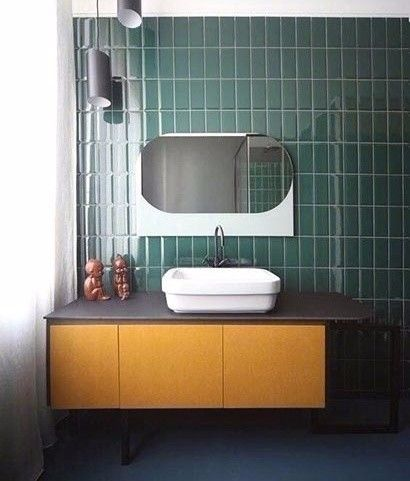选择好的瓷砖是成功装修卫生间的一半，瓷砖不仅装饰美化了卫生间，更是一个卫生间功能性好坏与否的主要体现。第一，选择瓷砖吸水率低的

一般来说，品质好的瓷砖，吸水率很低，这样能很快晾干。

若瓷砖没有注明吸水率，判断瓷砖吸水率高低的办法很简单，只要在样品瓷砖的背面滴上一滴水，看吸水性快慢，吸水性慢的较好。

吸水率高的瓷砖经热胀冷缩后会导致瓷砖表面龟裂及整块墙地砖剥落。第二，选择瓷砖质地密度高的

选购瓷砖的时候，可以从侧面观察砖面是否平整，是否出现粗细不均的针孔。

同时，可敲击瓷砖倾听声音是否清脆，声音越脆，表示瓷砖的质地密度高，硬度较佳。这样的瓷砖铺设到卫浴空间，既不容易损坏，也便于维护清洁。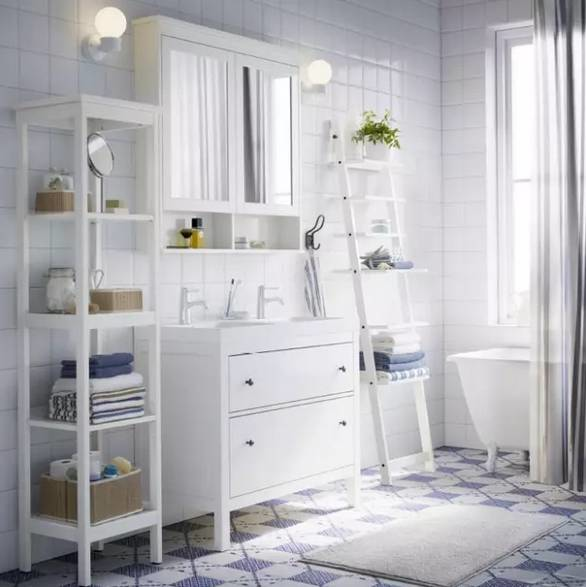第三，实现干湿分区

通过不同的瓷砖材质与花色品种，实现很好的划分，使两者互不干扰，避免以往那种水花四溅，洗浴后东抹西擦的，直接擦湿区。

这个国外比较常见的一种装修方法。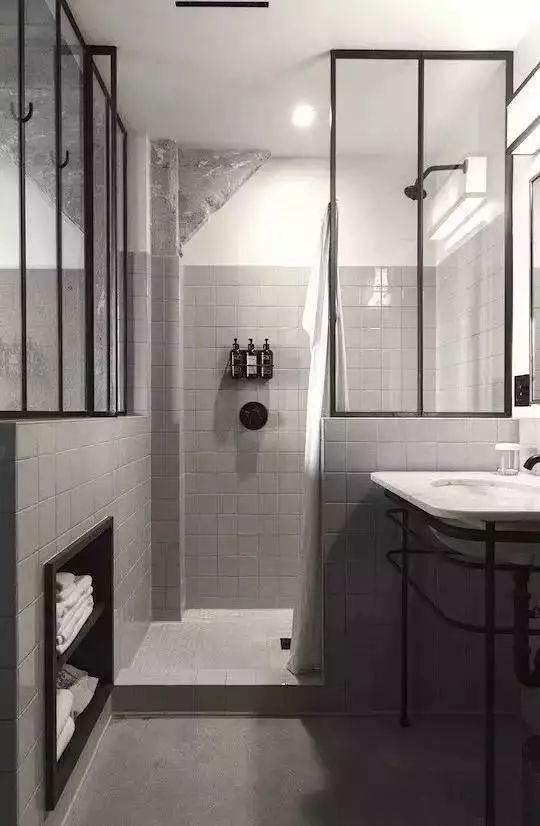第四，防滑

选择地面瓷砖的时候，一定是要采用防滑质地的，哑光面或浅凸凹造型的地砖。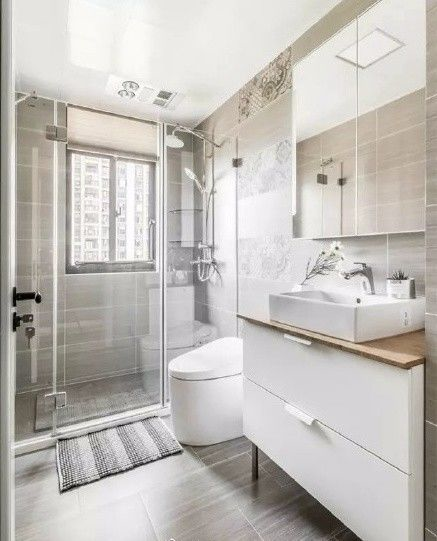第五，卫生间地砖vs墙砖的区分

卫生间地砖选购：

卫生间长期处于潮湿状态，地砖一定要保证防滑、防水，卫生间地砖一般选用通体砖，通体砖的防滑耐磨性较好；

地面常用的规格有300×300mm和330×330mm的。卫浴空间通常在2㎡至4㎡，即便卫浴空间面积较大，也尽量不要使用规格大的地砖。因为卫浴空间相对居室空间小，转角也多，太大的瓷砖切割频率高，浪费较大，也不便装地漏，不便地面找平，造成浪费。卫生间墙砖选购：

一般选用釉面砖，釉面砖色彩图案丰富，防污能力强。在家庭最常用的是釉面砖，通行的规格有300×400mm、300×300mm、250×330mm。墙面瓷砖的规格一般以实际的空间大小来确定。墙面砖的选择上可以使用亮光砖，也可以使用亚光砖。

第六，除了质量要好，好看也还是要的。

时尚界有小白鞋，装修界有小白砖。无论大空间还是小空间小白砖都能带来一种简洁明亮、干净质朴的感觉。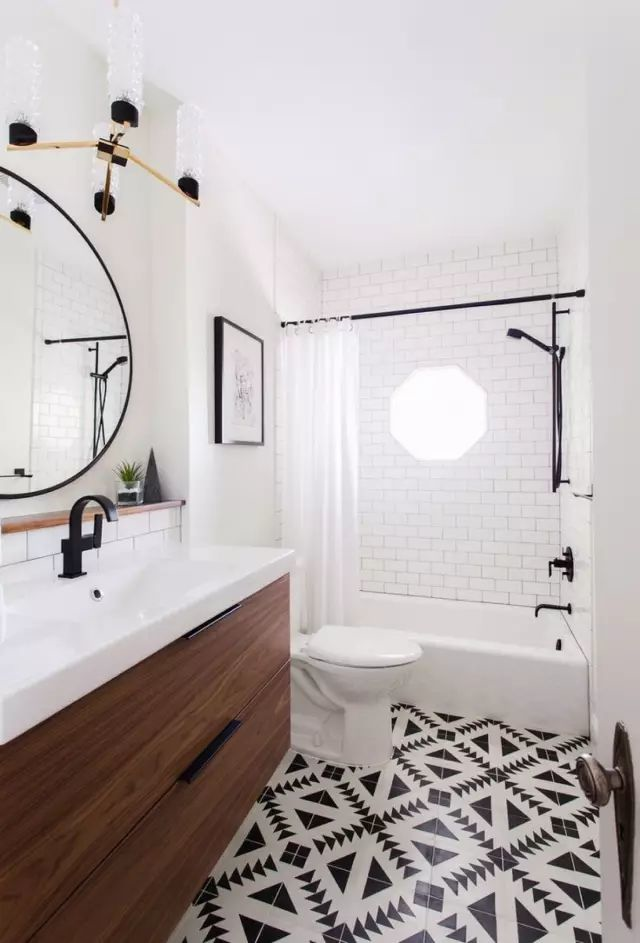跻身成为“国民建材”，受到越来越多人的宠爱，无敌百搭，简简单单一小块，却可以装点出的让人惊艳空间效果。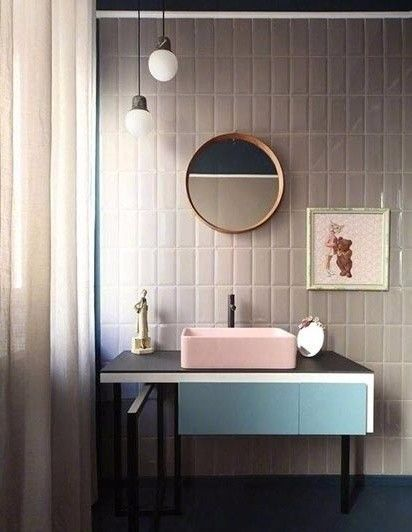如果你的卫生间采光很好，也能保证卫生间充足的照明，那除了小白砖还有另外一个百搭的选择，灰色砖。

灰色砖是一种几乎不会出错的选择，好搭、耐脏，易出高级感。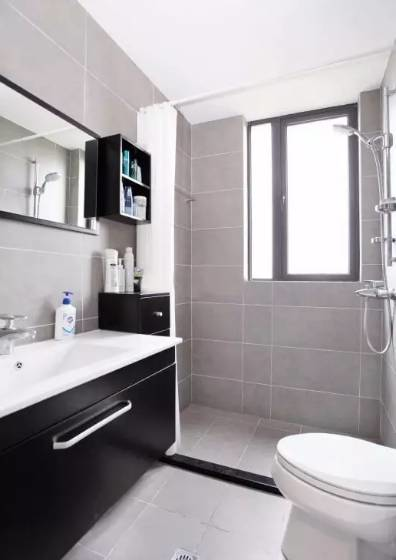不想卫生间太单调？

市面上的花砖也是一个不错的选择，也不用铺的满墙都是，局部点缀的手法会更出彩。▲多色拼砖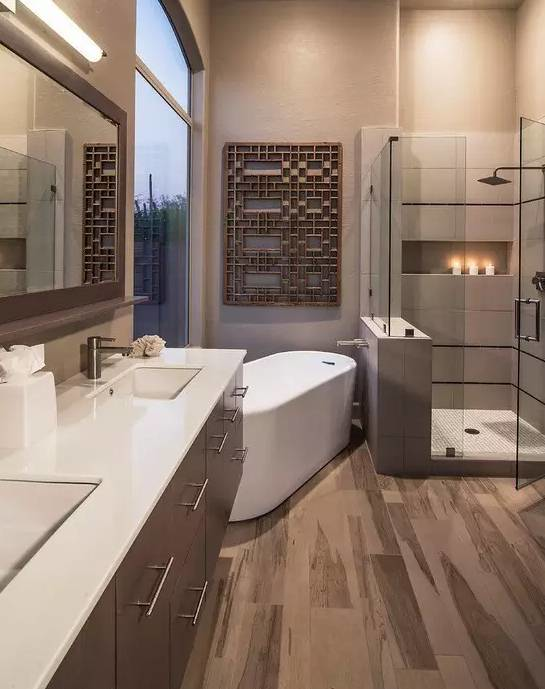▲木纹砖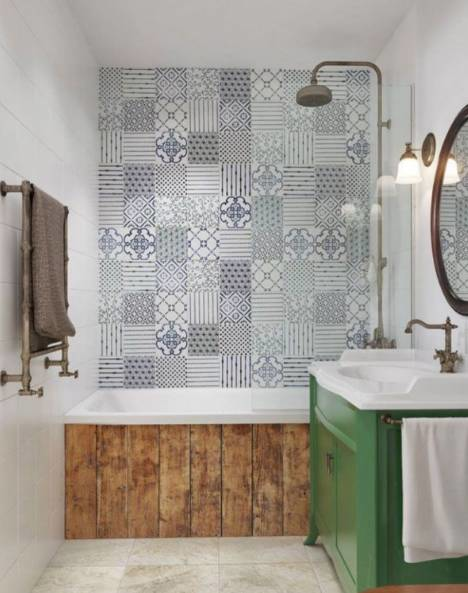▲铺在淋浴区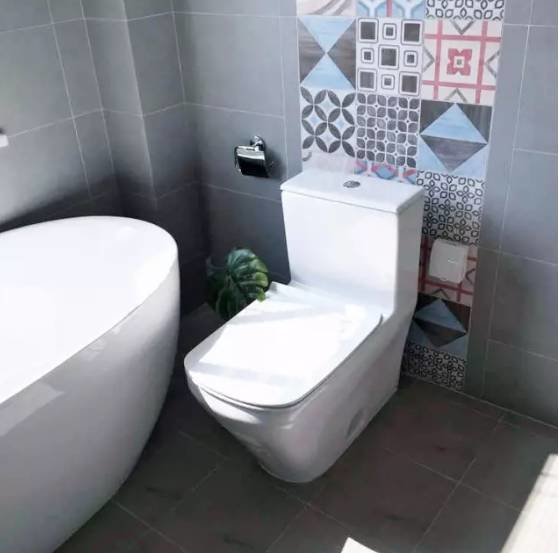▲铺在马桶后面

第七，根据面积的大小遵循几个大的原则：

1、大面积卫生间

大面积卫生间的瓷砖可以选用深色系，在中间搭配上浅色系的腰线或者在底部搭配浅色的踢脚线，能让整个空间不会太沉闷。

地砖也可选择和墙面瓷砖相同的颜色，但洁具要选用浅色系，这样整体效果才会显得尊贵大气。2、中等面积卫生间

中等面积的卫生间可以大面积运用暖色调瓷砖，这样装出的效果平实自然，看着会很舒心；

也可以小面积运用暖色调，冷色调加以搭配，会显得卫生间富有个性，不会太平庸。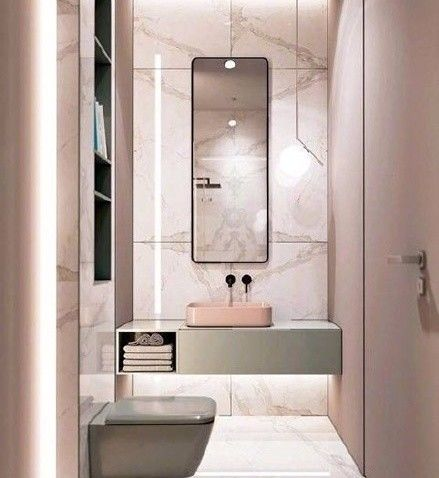3、小面积卫生间

面积小的卫生间最好选择浅色系的瓷砖，这样可以起到扩大空间的效果。

配深色的地砖，不会显得空间头重脚轻。好了，说了这么多，我要去领航装饰挑砖啦，他们有2000m⊃2;的材料展示厅，不用跑那么多建材市场就可以选到合适的瓷砖，而且都是工厂价直销，直接省去50%，真是省薪又省力！

`声明：本文由入驻焦点开放平台的作者撰写，除焦点官方账号外，观点仅代表作者本人，不代表焦点立场错误信息举报电话： 400-099-0099，邮箱：jubao@vip.sohu.com，或点此进行意见反馈，或点此进行举报投诉。`A B C D E F G H J K L M N P Q R S T W X Y Z
A - B - C - D - E
• A
• 鞍山
• 安庆
• 安阳
• 安顺
• 安康
• 澳门
• B
• 北京
• 保定
• 包头
• 巴彦淖尔
• 本溪
• 蚌埠
• 亳州
• 滨州
• 北海
• 百色
• 巴中
• 毕节
• 保山
• 宝鸡
• 白银
• 巴州
• C
• 承德
• 沧州
• 长治
• 赤峰
• 朝阳
• 长春
• 常州
• 滁州
• 池州
• 长沙
• 常德
• 郴州
• 潮州
• 崇左
• 重庆
• 成都
• 楚雄
• 昌都
• 慈溪
• 常熟
• D
• 大同
• 大连
• 丹东
• 大庆
• 东营
• 德州
• 东莞
• 德阳
• 达州
• 大理
• 德宏
• 定西
• 儋州
• 东平
• E
• 鄂尔多斯
• 鄂州
• 恩施
F - G - H - I - J
• F
• 抚顺
• 阜新
• 阜阳
• 福州
• 抚州
• 佛山
• 防城港
• G
• 赣州
• 广州
• 桂林
• 贵港
• 广元
• 广安
• 贵阳
• 固原
• H
• 邯郸
• 衡水
• 呼和浩特
• 呼伦贝尔
• 葫芦岛
• 哈尔滨
• 黑河
• 淮安
• 杭州
• 湖州
• 合肥
• 淮南
• 淮北
• 黄山
• 菏泽
• 鹤壁
• 黄石
• 黄冈
• 衡阳
• 怀化
• 惠州
• 河源
• 贺州
• 河池
• 海口
• 红河
• 汉中
• 海东
• 怀来
• I
• J
• 晋中
• 锦州
• 吉林
• 鸡西
• 佳木斯
• 嘉兴
• 金华
• 景德镇
• 九江
• 吉安
• 济南
• 济宁
• 焦作
• 荆门
• 荆州
• 江门
• 揭阳
• 金昌
• 酒泉
• 嘉峪关
K - L - M - N - P
• K
• 开封
• 昆明
• 昆山
• L
• 廊坊
• 临汾
• 辽阳
• 连云港
• 丽水
• 六安
• 龙岩
• 莱芜
• 临沂
• 聊城
• 洛阳
• 漯河
• 娄底
• 柳州
• 来宾
• 泸州
• 乐山
• 六盘水
• 丽江
• 临沧
• 拉萨
• 林芝
• 兰州
• 陇南
• M
• 牡丹江
• 马鞍山
• 茂名
• 梅州
• 绵阳
• 眉山
• N
• 南京
• 南通
• 宁波
• 南平
• 宁德
• 南昌
• 南阳
• 南宁
• 内江
• 南充
• P
• 盘锦
• 莆田
• 平顶山
• 濮阳
• 攀枝花
• 普洱
• 平凉
Q - R - S - T - W
• Q
• 秦皇岛
• 齐齐哈尔
• 衢州
• 泉州
• 青岛
• 清远
• 钦州
• 黔南
• 曲靖
• 庆阳
• R
• 日照
• 日喀则
• S
• 石家庄
• 沈阳
• 双鸭山
• 绥化
• 上海
• 苏州
• 宿迁
• 绍兴
• 宿州
• 三明
• 上饶
• 三门峡
• 商丘
• 十堰
• 随州
• 邵阳
• 韶关
• 深圳
• 汕头
• 汕尾
• 三亚
• 三沙
• 遂宁
• 山南
• 商洛
• 石嘴山
• T
• 天津
• 唐山
• 太原
• 通辽
• 铁岭
• 泰州
• 台州
• 铜陵
• 泰安
• 铜仁
• 铜川
• 天水
• 天门
• W
• 乌海
• 乌兰察布
• 无锡
• 温州
• 芜湖
• 潍坊
• 威海
• 武汉
• 梧州
• 渭南
• 武威
• 吴忠
• 乌鲁木齐
X - Y - Z
• X
• 邢台
• 徐州
• 宣城
• 厦门
• 新乡
• 许昌
• 信阳
• 襄阳
• 孝感
• 咸宁
• 湘潭
• 湘西
• 西双版纳
• 西安
• 咸阳
• 西宁
• 仙桃
• 西昌
• Y
• 运城
• 营口
• 盐城
• 扬州
• 鹰潭
• 宜春
• 烟台
• 宜昌
• 岳阳
• 益阳
• 永州
• 阳江
• 云浮
• 玉林
• 宜宾
• 雅安
• 玉溪
• 延安
• 榆林
• 银川
• Z
• 张家口
• 镇江
• 舟山
• 漳州
• 淄博
• 枣庄
• 郑州
• 周口
• 驻马店
• 株洲
• 张家界
• 珠海
• 湛江
• 肇庆
• 中山
• 自贡
• 资阳
• 遵义
• 昭通
• 张掖
• 中卫

1室1厅1厨1卫1阳台

1
2
3
4
5

0
1
2

1

1

0
1
2
3报名成功，资料已提交审核A B C D E F G H J K L M N P Q R S T W X Y Z
A - B - C - D - E
• A
• 鞍山
• 安庆
• 安阳
• 安顺
• 安康
• 澳门
• B
• 北京
• 保定
• 包头
• 巴彦淖尔
• 本溪
• 蚌埠
• 亳州
• 滨州
• 北海
• 百色
• 巴中
• 毕节
• 保山
• 宝鸡
• 白银
• 巴州
• C
• 承德
• 沧州
• 长治
• 赤峰
• 朝阳
• 长春
• 常州
• 滁州
• 池州
• 长沙
• 常德
• 郴州
• 潮州
• 崇左
• 重庆
• 成都
• 楚雄
• 昌都
• 慈溪
• 常熟
• D
• 大同
• 大连
• 丹东
• 大庆
• 东营
• 德州
• 东莞
• 德阳
• 达州
• 大理
• 德宏
• 定西
• 儋州
• 东平
• E
• 鄂尔多斯
• 鄂州
• 恩施
F - G - H - I - J
• F
• 抚顺
• 阜新
• 阜阳
• 福州
• 抚州
• 佛山
• 防城港
• G
• 赣州
• 广州
• 桂林
• 贵港
• 广元
• 广安
• 贵阳
• 固原
• H
• 邯郸
• 衡水
• 呼和浩特
• 呼伦贝尔
• 葫芦岛
• 哈尔滨
• 黑河
• 淮安
• 杭州
• 湖州
• 合肥
• 淮南
• 淮北
• 黄山
• 菏泽
• 鹤壁
• 黄石
• 黄冈
• 衡阳
• 怀化
• 惠州
• 河源
• 贺州
• 河池
• 海口
• 红河
• 汉中
• 海东
• 怀来
• I
• J
• 晋中
• 锦州
• 吉林
• 鸡西
• 佳木斯
• 嘉兴
• 金华
• 景德镇
• 九江
• 吉安
• 济南
• 济宁
• 焦作
• 荆门
• 荆州
• 江门
• 揭阳
• 金昌
• 酒泉
• 嘉峪关
K - L - M - N - P
• K
• 开封
• 昆明
• 昆山
• L
• 廊坊
• 临汾
• 辽阳
• 连云港
• 丽水
• 六安
• 龙岩
• 莱芜
• 临沂
• 聊城
• 洛阳
• 漯河
• 娄底
• 柳州
• 来宾
• 泸州
• 乐山
• 六盘水
• 丽江
• 临沧
• 拉萨
• 林芝
• 兰州
• 陇南
• M
• 牡丹江
• 马鞍山
• 茂名
• 梅州
• 绵阳
• 眉山
• N
• 南京
• 南通
• 宁波
• 南平
• 宁德
• 南昌
• 南阳
• 南宁
• 内江
• 南充
• P
• 盘锦
• 莆田
• 平顶山
• 濮阳
• 攀枝花
• 普洱
• 平凉
Q - R - S - T - W
• Q
• 秦皇岛
• 齐齐哈尔
• 衢州
• 泉州
• 青岛
• 清远
• 钦州
• 黔南
• 曲靖
• 庆阳
• R
• 日照
• 日喀则
• S
• 石家庄
• 沈阳
• 双鸭山
• 绥化
• 上海
• 苏州
• 宿迁
• 绍兴
• 宿州
• 三明
• 上饶
• 三门峡
• 商丘
• 十堰
• 随州
• 邵阳
• 韶关
• 深圳
• 汕头
• 汕尾
• 三亚
• 三沙
• 遂宁
• 山南
• 商洛
• 石嘴山
• T
• 天津
• 唐山
• 太原
• 通辽
• 铁岭
• 泰州
• 台州
• 铜陵
• 泰安
• 铜仁
• 铜川
• 天水
• 天门
• W
• 乌海
• 乌兰察布
• 无锡
• 温州
• 芜湖
• 潍坊
• 威海
• 武汉
• 梧州
• 渭南
• 武威
• 吴忠
• 乌鲁木齐
X - Y - Z
• X
• 邢台
• 徐州
• 宣城
• 厦门
• 新乡
• 许昌
• 信阳
• 襄阳
• 孝感
• 咸宁
• 湘潭
• 湘西
• 西双版纳
• 西安
• 咸阳
• 西宁
• 仙桃
• 西昌
• Y
• 运城
• 营口
• 盐城
• 扬州
• 鹰潭
• 宜春
• 烟台
• 宜昌
• 岳阳
• 益阳
• 永州
• 阳江
• 云浮
• 玉林
• 宜宾
• 雅安
• 玉溪
• 延安
• 榆林
• 银川
• Z
• 张家口
• 镇江
• 舟山
• 漳州
• 淄博
• 枣庄
• 郑州
• 周口
• 驻马店
• 株洲
• 张家界
• 珠海
• 湛江
• 肇庆
• 中山
• 自贡
• 资阳
• 遵义
• 昭通
• 张掖
• 中卫• 手机• 分享
• 设计
免费设计
• 计算器
装修计算器
• 入驻
合作入驻
• 联系
联系我们
• 置顶
返回顶部